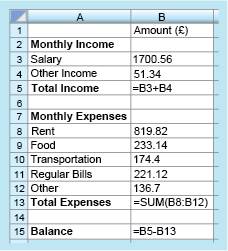Succeed with maths: part 2

Start this free course now. Just create an account and sign in. Enrol and complete the course for a free statement of participation or digital badge if available.

# 1.1 Finding the balance

A similar approach can be used for working out other values in the spreadsheet. Have a go yourself in this next activity.

## Activity _unit5.1.2 Activity 2 Working out the balance

Timing: Allow approximately 5 minutes

Formulas have also been used to calculate the total monthly expenses and the balance. If you were working these calculations out by hand, what would you do? Write this using the appropriate cell numbers and maths operators as shown in the previous example. Finally, check these comparing your answers using the formulas with the values in cell B13 and B15.

To calculate the total monthly expenses, you need to add the individual expenses of ‘Rent’, ‘Food’, ‘Transportation’, ‘Regular Bills’, and ‘Other’. The formula will therefore be:

To find the balance, you need to take the expenses away from the total income. So, the formula to calculate the balance will be:

Checking these now:

Comparing these with the values in the spreadsheet, it is clear that they agree. This should give us confidence that the formulas built are correct!

How are these formulas actually put into the spreadsheet though? To do this you type the formula directly into the relevant cell, starting with an equals sign to tell the software that you are entering a formula rather than a word. This is shown in Figure 2:Figure _unit5.1.2 Figure 2 Finding the balance

Notice that in cell B13, a shorthand form for the sum (total) has been entered. The formula ‘= B8 + B9 + B10 + B11 + B12’ could have also been entered, but it is much quicker to use the shorthand form ‘= SUM(B8:B12)’, which is shown here. This instruction tells the computer to add the values in all the cells from B8 to B12.

If you have access to spreadsheet software, try and recreate this example now and see how you get on with entering formulas yourself.

Another advantage of using a spreadsheet is that if you change some of the numbers, all the calculations that use that particular number will be automatically updated to reflect the change. For example, in this budget, the amounts for the salary, rent and the regular bills are likely to remain the same from one month to the next, and may only be updated once or twice a year. However, the amounts for food, transportation, other bills and other income will probably change from month to month. These values can be changed easily on the spreadsheet and the revised balance produced immediately, taking some of the work from you and reducing the risk of calculation errors.